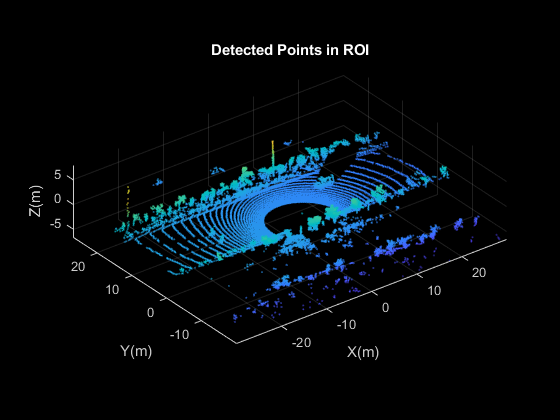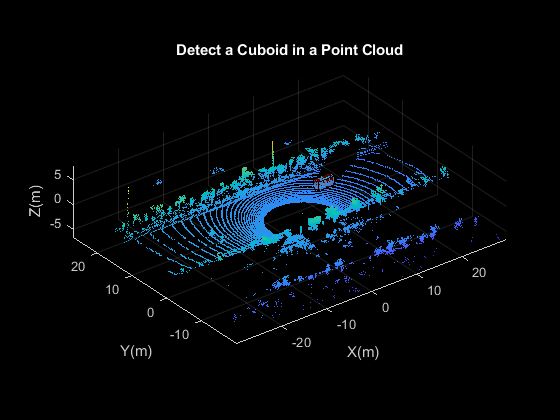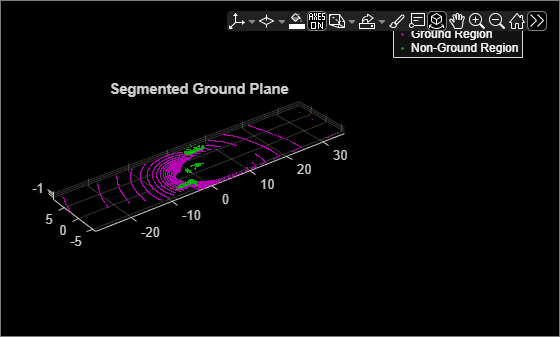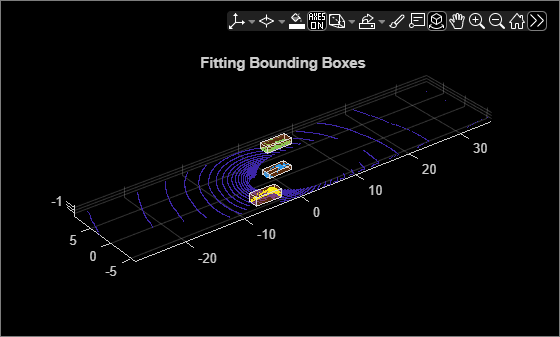# cuboidModel

Parametric cuboid model

Since R2020b

## Description

The `cuboidModel` object stores the parameters of a parametric cuboid model. After you create a `cuboidModel` object, you can extract cuboid corner points, and points within the cuboid using the object functions. Cuboid models are used to store the output of `pcfitcuboid` function. It is a shape fitting function which fits a cuboid over a point cloud.

## Creation

There are two ways to create a `cuboidModel` object.

• Create a cuboid model by specifying the cuboid parameters in the `cuboidModel` function.

• Fit a cuboid model over a point cloud using the `pcfitcuboid` function.

### Description

example

````model = cuboidModel(params)` constructs a parametric cuboid model from the 1-by-9 input vector, `params`.```
````model = pcfitcuboid(ptCloudIn)` fits a cuboid over the input point cloud data. The `pcfitcuboid` function stores the properties of the cuboid in a parametric cuboid model object, `model`.```

example

````model = pcfitcuboid(ptCloudIn,indices)` fits a cuboid over a selected set of points, `indices`, in the input point cloud.```

## Properties

expand all

Cuboid model parameters, stored as a nine-element row vector of the form [xctr yctr zctr xlen ylen zlen xrot yrot zrot].

• xctr, yctr, and zctr specify the center of the cuboid.

• xlen, ylen, and zlen specify the length of the cuboid along the x-, y-, and z-axis, respectively, before rotation has been applied.

• xrot, yrot, and zrot specify the rotation angles in degrees for the cuboid along the x-, y-, and z-axis, respectively. These angles are clockwise-positive when looking in the forward direction of their corresponding axes.

The figure shows how these values determine the position of a cuboid.These parameters are specified by the `params` input argument.

Data Types: `single` | `double`

Center of the cuboid, stored as a three-element row vector of the form [xctr yctr zctr]. The vector contains the 3-D coordinates of the cuboid center in the x-, y-, and z-axis, respectively.

This property is derived from the `Parameters` property.

Data Types: `single` | `double`

Dimensions of the cuboid, stored as a three-element row vector of the form [xlen ylen zlen]. The vector contains the length of the cuboid along the x-, y-, and z-axis, respectively.

This property is derived from the `Parameters` property.

Data Types: `single` | `double`

Orientation of the cuboid, stored as a three-element row vector of the form, [xrot yrot zrot], in degrees. The vector contains the rotation of the cuboid along the x-, y-, and z-axis, respectively.

This property is derived from the `Parameters` property.

If the orientation is in quaternion, convert the quaternion to Euler angles in degrees to create a cuboid model.

Data Types: `single` | `double`

## Object Functions

 `getCornerPoints` Get corner points of cuboid model `findPointsInsideCuboid` Find points enclosed by cuboid model `plot` Plot cuboid model

## Examples

collapse all

Detect a cuboid in a point cloud using `pcfitcuboid` function. The function stores the cuboid parameters as a `cuboidModel` object.

Read point cloud data into the workspace.

`ptCloud = pcread('highwayScene.pcd');`

Search the point cloud within a specified region of interest (ROI). Create a point cloud of only the detected points.

```roi = [-30 30 -20 30 -8 13]; in = findPointsInROI(ptCloud,roi); ptCloudIn = select(ptCloud,in);```

Plot the point cloud of detected points.

``` figure pcshow(ptCloudIn.Location) xlabel('X(m)') ylabel('Y(m)') zlabel('Z(m)') title('Detected Points in ROI')```Find the indices of the points in a specified ROI within the point cloud.

```roi = [9.6 13.8 7.9 9.3 -2.5 3]; sampleIndices = findPointsInROI(ptCloudIn,roi);```

Fit a cuboid to the selected set of points in the point cloud.

``` model = pcfitcuboid(ptCloudIn,sampleIndices); figure pcshow(ptCloudIn.Location) xlabel('X(m)') ylabel('Y(m)') zlabel('Z(m)') title('Detect a Cuboid in a Point Cloud')```

Plot the cuboid box in the point cloud.

```hold on plot(model)```Display the internal properties of the `cuboidModel` object.

`model`
```model = cuboidModel with properties: Parameters: [11.4873 8.5997 -1.6138 3.6713 1.3220 1.7576 0 0 0.9999] Center: [11.4873 8.5997 -1.6138] Dimensions: [3.6713 1.3220 1.7576] Orientation: [0 0 0.9999] ```

Fit cuboid bounding boxes around clusters in a point cloud.

Load the point cloud data into the workspace.

`data = load('drivingLidarPoints.mat');`

Define and crop a region of interest (ROI) from the point cloud. Visualize the selected ROI of the point cloud.

```roi = [-40 40 -6 9 -2 1]; in = findPointsInROI(data.ptCloud,roi); ptCloudIn = select(data.ptCloud,in); hcluster = figure; panel = uipanel('Parent',hcluster,'BackgroundColor',[0 0 0]); ax = axes('Parent',panel,'Color',[0 0 0]); pcshow(ptCloudIn,'MarkerSize',30,'Parent',ax) title('Input Point Cloud')```Segment the ground plane. Visualize the segmented ground plane.

```maxDistance = 0.3; referenceVector = [0 0 1]; [~,inliers,outliers] = pcfitplane(ptCloudIn,maxDistance,referenceVector); ptCloudWithoutGround = select(ptCloudIn,outliers,'OutputSize','full'); hSegment = figure; panel = uipanel('Parent',hSegment,'BackgroundColor',[0 0 0]); ax = axes('Parent',panel,'Color',[0 0 0]); pcshowpair(ptCloudIn,ptCloudWithoutGround,'Parent',ax) legend('Ground Region','Non-Ground Region','TextColor', [1 1 1]) title('Segmented Ground Plane')```Segment the non-ground region of the point cloud into clusters. Visualize the segmented point cloud.

```distThreshold = 1; [labels,numClusters] = pcsegdist(ptCloudWithoutGround,distThreshold); labelColorIndex = labels; hCuboid = figure; panel = uipanel('Parent',hCuboid,'BackgroundColor',[0 0 0]); ax = axes('Parent',panel,'Color',[0 0 0]); pcshow(ptCloudIn.Location,labelColorIndex,'Parent',ax) title('Fitting Bounding Boxes') hold on```

Fit bounding box on each cluster, visualized as orange highlights.

```for i = 1:numClusters idx = find(labels == i); model = pcfitcuboid(ptCloudWithoutGround,idx); plot(model) end```## Version History

Introduced in R2020b